### Conditional Simulation

For a conditional simulation, this distribution of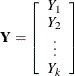must be conditioned on the values of the CONDITION variables. The relevant general result concerning conditional distributions of multivariate normal random variables is the following. Let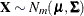, where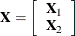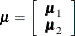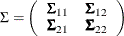and where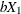is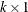,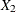is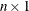,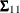is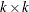,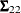is, and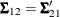is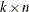, with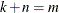. The full vectorhas simply been partitioned into two subvectors,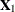and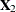, and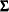has been similarly partitioned into covariances and cross covariances.

With this notation, the distribution ofconditioned on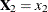is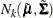, with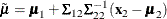and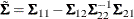See Searle (1971, pp. 46–47) for details.

Using the SIMNORMAL procedure corresponds with the conditional simulation as follows. Let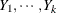be the VAR variables as before (k is the number of variables in the VAR list). Let the mean vector for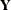be denoted by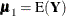. Let the CONDITION variables be denoted by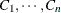(where n is the number of variables in the COND list). Let the mean vector for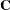be denoted by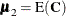and the conditioning values be denoted by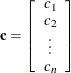Then stacking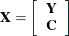the variance ofis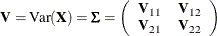where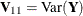,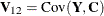, and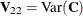. By using the preceding general result, the relevant covariance matrix is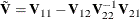and the mean is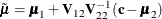By using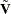and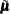, simulating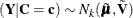now proceeds as in the unconditional case.